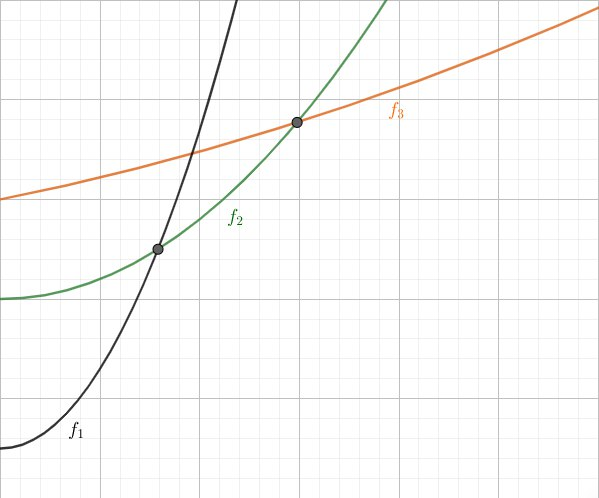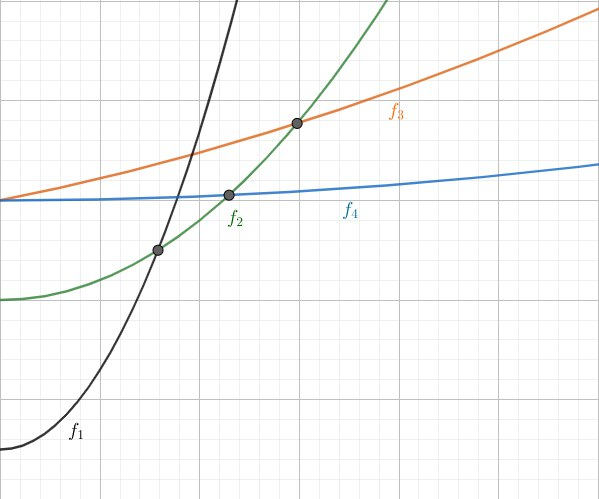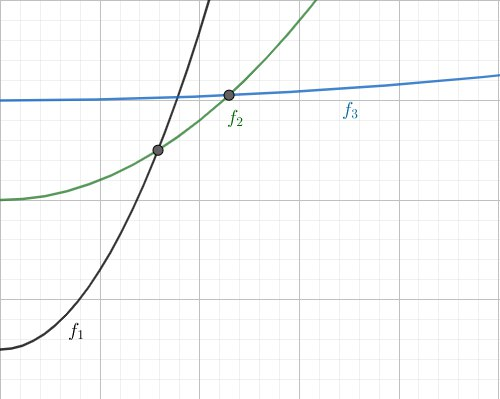# POLY - Editorial

Author: Trung Nguyen
Tester: Istvan Nagy
Editorialist: Oleksandr Kulkov

HARD

### PREREQUISITES:

Li Chao segment tree OR good sense of lower hull trick

### PROBLEM:

You have n polynomials y_i(x)=a_0+a_1x+a_2x^2+a_3x^3 and q queries in which you are asked to find y_i which minimizes y_i(t).

### QUICK EXPLANATION:

Solve problem via brute force for small t and for large use Li Chao segment tree.

### EXPLANATION:

Consider polynomial y_i-y_j for some i and j. Its degree is at most three.

Lemma: Polynomial y=x^3+ax^2+bx+c has at most one root greater than k=\sqrt{\max(|b|,|c|)}+2.

Proof: Let u\geq v >k\geq w to be the roots of y. Then y=(x-u)(x-v)(x-w) so b=uv+uw+vw, c=-uvw. Since u,v>\sqrt {|c|} it holds that |w|<1. Thus b=uv+w(u+v)> uv-(u+v)=(u-1)(v-1)-1. But since u,v>\sqrt {|b|} + 2 we have b> (\sqrt {|b|} + 1)^2-1=|b|+2\sqrt {|b|}>b which is contradiction.

\tag*{$\Box$}

Given this and the fact that for x^2+bx+c at most one root greater than \sqrt{|c|} (due to their product being equal to c) we can just manually check first 350>\sqrt{10^5} integer points and consider functions only on set of points after 350 when any two functions will have at most one intersection points due to their difference being at most third degree polynomial.

Now to handle queries on [350,+\infty) we will use Li Chao segment tree. Assume you’re given set of functions such that each two can intersect at most once. Let’s keep in each vertex of segment tree some function in such way that if we go from root to the leaf, it will be guaranteed that among functions we meet on the path will be the one giving minimum value in that leaf. Let’s see how to construct it.

Assume we’re in some vertex corresponding to half-segment [l;r) and function f_{old} is kept there and we adding to the set function f_{new}. Then intersection point will be either in [l;m) or in [m;r) where m=\left\lfloor\dfrac{l+r}{2}\right\rfloor. We can efficiently learn that comparing values of functions in points l and m. If dominating function changes then it’s in [l;m) otherwise it’s in [m;r). Now for the half of segment where there no intersection we pick lower function and write it in current vertex. After that we recursively go to the other half of segment with the function which was upper one. As you can see this will keep correctness on the first half of segment and in the other one correctness will be maintained during the recursive call. Thus we can add functions and check the minimum value in the point in O(\log C).

Here is the illustration of what’s going on in the vertex when we consider adding in it new function:

In this particular case we will keep new in the vertex and recursively call in the left segment with old function.

### ALTERNATIVE SOLUTION

One can construct hull of functions in offline instead of using LiChao tree. If function f_a less than function f_b on x\to+\infty and they have at most one point of intersection then f_a coefficients (a_3,a_2,a_1,a_0) lexicographically smaller than coefficients of f_b. Thus we can firstly sort functions in descending order of their coefficients and then add functions one by one to maintain the lower envelope.

We will keep them in stack. Assume this stack to be (f_1,f_2,\dots,f_k) and points 350< x_2< \dots< x_k are the intersections of the functions. Then function f_i have least value among all functions on (x_i,x_{i+1}) with x_1=350,x_{k+1}=+\infty. If we consider new function f_{k+1} with all coefficients lexicographically smaller than ones of functions we have then there will be such point x_0 that it will have smaller values than all functions on (x_0,+\infty) and it will still have larger values than functions from envelope on (350,x_0). So we can repeatedly remove functions f_k till they have larger values than both f_{k+1} and f_{k-1} at the point where functions f_{k-1} and f_{k+1} intersect and add function f_{k+1} in the stack afterwards. This will be sufficient to build lower envelope of functions and if you also keep their intersection points then you can use binary search to find function with lowest value in given point x.

This approach strongly generalizes well-known convex hull trick for the case in which we have set of non-necessarily convex functions with the property that they have at most one intersection and we can calculate beforehand which order will they have if we consider them for x\to+\infty.

Finally, to illustrate the solution, I’ll provide some pictures. Assume you had functions f_1, f_2, f_3 in the stack.And now you want to add f_4.As we see function f_3 is no use at all since it is above point of intersection of functions f_2 and f_4. So we remove it and place f_4 in its place in the stack.### AUTHOR’S AND TESTER’S SOLUTIONS:

Author’s solution can be found here.

Tester’s solution can be found here.

### RELATED PROBLEMS:

2 Likes

For the lower convex hull trick, why is assuming that each pair of functions intersect in at most one point correct?

@amr_keleg
Because they are increasing functions,so two lines will intersect atmost 1 time(provided both are not the same function)

Edit: Not necessary unless linear as nilesh specified,however beyond a particular x it intersects atmost 1.

Read the Lemma and 1st paragraph. there would be at most 1 intersection point past 350.

You are wrong. This would only be true when rate of increase is constant i.e. the functions are linear.

1 Like

Ok , I see.
Yet I have another two questions.

1. Is there a formula to find the largest real root of a cubic equation?
Should I use binary Search (bisection method) or other numerical methods?
2. Does this lemma somehow generalise to higher order polynomials?

@nilesh3105
Yeah i got it!!.
Thanx for correcting me.

Any resource on Lichao_SegmentTree ? I read the CF comment already, but was not enough for me to come up with this magic Data Structure, i will be glad if anyone can help1 Like